causal inference

Some notes on causal inference both from the following resources:

# basics

• confounding = difference between groups other than the treatment which affects the response
• this is the key problem when using observational (non-experimental) data to make causal inferences
• problem occurs because we don’t get to see counterfactuals
• ex from Pearl where Age is the confounder• potential outcomes = counterfactual outcomes $Y^{t=1}, Y^{t=0}$
• treatment = intervention, exposure, action
• potential outcomes are often alternatively written as $Y(1)$ and $Y(0$) or $Y_1$ and $Y_0$
•  alternatively, $P(Y=y do(T=1))$ and $P(Y=y do(T=0))$ or $P(Y=y set(T=1))$ and $P(Y=y set(T=0))$
• treatment $T$ and outcome $Y$ (from “What If”):
•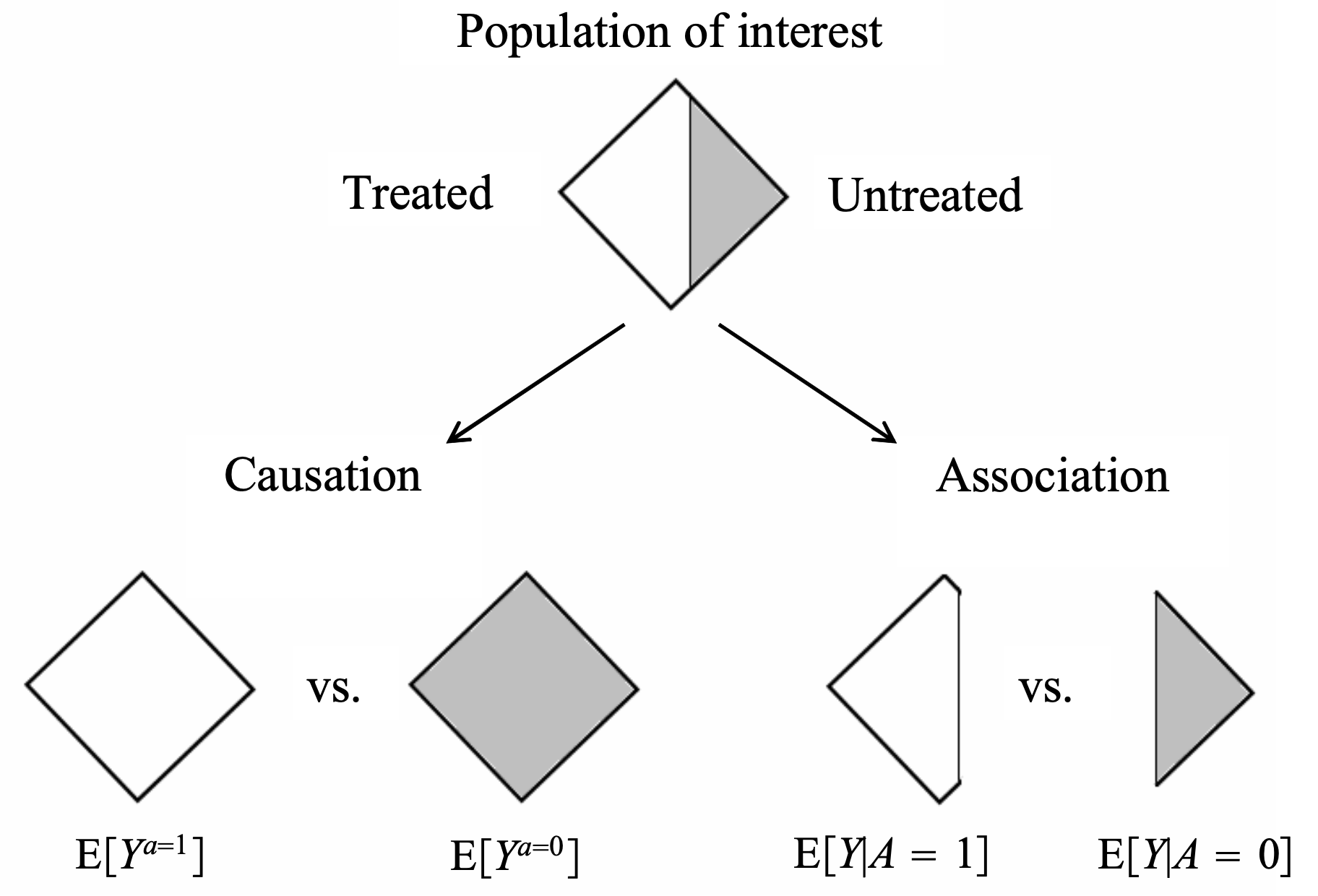• different approaches to causal analysis
• experimental design: collect data in a way that enables causal conclusions
• ex. randomized control trial (RCT) - controls for any possible confounders
• quasi-experiments: without explicit random assignment, some data pecularity approximates randomization
• ex. regression discontinuity analysis
• ex. instrumental variables - variable which can be used to effectively due a RCT because it was made random by some external factor
• post-hoc analysis: by arguing that certain assumptions hold, we can draw causal conclusions from non-observational data
• ex. regression-based adjustment after assuming ignorability
• some assumptions are not checkable, and we can only reason about how badly they can go wrong (e.g. using sensitivity analysis)
• background
• very hard to decide what to include and what is irrelevant
• epiphenomenon - a correlated effect (not a cause)
• a secondary effect or byproduct that arises from but does not causally influence a process
• ontology - study of being, concepts, categories
• nodes in graphs must refer to stable concepts
• ontologies are not always stable
• world changes over time
• “looping effect” - social categories (like race) are constantly chainging because people who putatively fall into such categories to change their behavior in possibly unexpected ways
• epistemology - theory of knowledge
• clear distinction between identification and estimation (and third problem is discovery - what is the structure?)
• a causal quantity is identifiable if we can compute it from a purely statistical quantity
•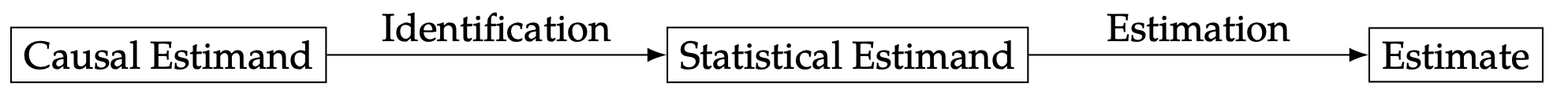## intuition

• bradford hill criteria - some simple criteria for establishing causality (e.g. strength, consistency, specificity)
• association is circumstantial evidence for causation
• no causation without manipulation (rubin, 1975; Holland, 1986)
• in this manner, something like causal effect of race/gender doesn’t make sense
• can partially get around this by changing race $\to$ perceived race
• weaker view (e.g. of Pearl) is that we only need to be able to understand how entities interact (e.g. write an SEM)
• different levels
• experiment: experiment, RCT, natural experiment, observation
• evidence: marginal correlation, regression, invariance, causal
• inference (pearl’s ladder of causality): prediction/association, intervention, counterfactuals
• kosuke imai’s levels of inference: descriptive, predictive, causal

## measures of association

• correlation
• regression coefficient
•  risk difference = $P(Y=1 T=1) - P(Y=1 T=0)$
•  risk ratio = relative risk = $P(Y=1 T=1) / P(Y=1 T=0)$
•  odds ratio = $\frac{P(Y=1 T=1) / P(Y=0 T=1)}{P(Y=1 T=0) / P(Y=0 T=0)}$
• measures association (1 is independent, >1 is positive association, <1 is negative association)
• odds that $P(Y=1)$ = $P(Y=1)/P(Y \neq 1)$

## causal ladder (different levels of inference)

1.  prediction/association $P(Y T)$
• only requires joint distr. of the variables
1.  intervention $P(Y^{T=t}) = P(Y do(T=t))$
• we can change things and get conditionals based on evidence after intervention
• represents the conditional distr. we would get if we were to manipulate $t$ in a randomized trial
• to get this, we assume the causal structure (can still kind of test it based on conditional distrs.)
• having assumed the structure, we delete all edges going into a do operator and set the value of $x$
•  then, do-calculus yields a formula to estimate $p(y do(x))$ assuming this causal structure
• see introductory paper here, more detailed paper here (pearl 2013)
• by assuming structure, we learn how large impacts are
1.  counterfactuals $P(Y^{T=t’} = y’ T=t, y=y)$
• we can change things and get conditionals based on evidence before intervention

•  instead of intervention $p(y do(x))$ we get $p(y^* x^, u=u)$ where $u$ represents fixing all the other variables and $y^$ and $x^*$ are not observed
•  averaging over all data points, we’d expect to get something similar to the intervention $p(y do(x))$
• probabalistic answer to a “what would have happened if” question
• e.g. “Given that Hillary lost and didn’t visit Michigan, would she win if she had visited Michigan?”
• e.g. “What fraction of patients who are treated and died would have survived if they were not treated?”
• this allows for our intervention to contradict something we condition on
• simple matching is often not sufficient (need a very good model for how to match, hopefully a causal one)
• key difference with standard intervention is that we incorporate available evidence into our calculation
• available evidence influences exogenous variables
• this is for a specific data point, not a randomly sampled data point like an intervention would be
• requires SEM, not just causal graph

# frameworks

## fisher randomization test

• this framework seeks evidence against the null hypothesis (e.g. that there is no causal effect)

• fisher null hypothesis: $H_{0F}: Y_i^{T=0} = Y_i^{T=1}\quad \forall i$
• also called strong null hypothesis = sharp null hypothesis (Rubin, 1980)
• weak null hypothesis would be $\bar Y_i^{T=0} = Y_i^{T=1}$
• can work for any test statistic $test$
• only randomness comes from treatment variable - this allows us to get randomization distribution for a test-statistic $test(T, Y^{T=1}, Y^{T=0})$
• this yields $p$-values: $p= \frac 1 M \sum_{m=1}^M \mathbb 1 { test(\mathbf t^m, \mathbf Y) \geq test (\mathbf T, \mathbf Y) }$
• can approximate this with Monte Carlo permutation test, with $R$ random permutations of $\mathbf T$: $p= \frac 1 R \sum_r \mathbb 1 { test(\mathbf t^r, \mathbf Y) \geq test (\mathbf T, \mathbf Y) }$
• canonical choices of test-statistic
• difference in means: $\hat \tau = \hat{\bar{Y}}^{T=1} - \hat{\bar{Y}}^{T=0}$
• $= \frac 1 {n_1} \sum_i T_i Y_i - \frac 1 {n_0} \sum_i (1 - T_i) Y_i$
• $= \frac 1 {n_1} \sum_{T_i=1} Y_i - \frac 1 {n_0} \sum_{T_i=0} Y_i$
• studentized statistic: $t=\frac{\hat \tau}{\sqrt{\frac{\hat S^2(T=1)}{n_1}+\frac{\hat S^2 (T=0)}{n_0}}}$
• allows for variance to change between groups (heteroscedasticity)
• wilcoxon rank sum: $W = \sum_i T_i R_i$, where $R_i = #{j : Y_j \leq Y_i }$ is the rank of $Y_i$ in the observed samples
• the sum of the ranks is $n(n+1)/2$, and the mean of $W$ is $n_1(n+1)/2$
• less sensitive to outliers
•  kolmogorov-smirnov statistic: $D = \max_y \hat F_1(y) - \hat F_0 (y)$ where $\hat F_1(y) = \frac 1 {n_1} \sum_i T_i 1(Y_i \leq y)$, $\hat F_0(y) = \frac 1 {n_0} \sum_i (1 -T_i) 1(Y_i \leq y)$
• measures distance between distributions of treatment outcomes and control outcomes
• alternative neyman-pearson framework has a null + alternative hypothesis
• null is favored unless there is strong evidence to refute it

## potential outcome framework (neyman-rubin)

In this framework, try to explicitly compute the effect

• average treatment effect ATE $\tau = E {Y^{T=1} - Y^{T=0} }$
• individual treatment effect $\Delta = Y_i^{T=1} - Y_i^{T=0}$
•  conditional average effect $= E{Y^{T=1} - Y^{T=0}} X$
• estimator $\hat \tau = \hat{\bar{Y}}^{T=1} - \hat{\bar{Y}}^{T=0}$
• unbiased: $E(\hat \tau) = \tau$
• $V(\hat \tau) = \underbrace{S^2(1) / n_1 + S^2(0)/n_0}_{\hat V(\tau) \text{ conservative estimator}} - S^2(\tau)/n$
• 95% CI: $\hat \tau \pm 1.96 \sqrt{\hat V}$ (based on normal approximation)
• we could similarly get a p-value testing whether $\hat \tau$ goes to 0, unclear if this is better
• key assumptions: SUTVA, consistency, ignorability
• strict randomization framework: only assume treatment assignment is take to be a random variable
• alternatively assume population distr. from which potential outcomes are drawn
• advantages over DAGs: easy to express some common assumptions, such as monotonicity / convexity
1. neyman-rubin model: $Y_i = \begin{cases} Y_i^{T=1}, &\text{if } T_i=1\Y_i^{T=0}, &\text{if } T_i=0 \end{cases}$
• equivalently, $Y_i = T_i Y_i^{T=1} + (1-T_i) Y_i^{T=0}$ - $\widehat{ATE} = \mathbb E [\hat{Y}^{T=1} - \hat{Y}^{T=0}]$ - $\widehat{ATE}_{adj} = [\bar{a}_A - (\bar{x}_A - \bar{x})^T \hat{\theta}_A] - [\bar{b}_B - (\bar{x}_B - \bar{x})^T \hat{\theta}_B]$
• $\hat{\theta}A = \mathrm{argmin} \sum{i \in A} (a_i - \bar{a}_A - (x_i - \bar{x}_A)^T \theta)^2$
2. neyman-pearson
• null + alternative hypothesis
3. can also take a bayesian perspective on the missing data

## DAGs / structural causal models (pearl et al.)

In this framework, depict assumptions in a DAG / SEM and use it to reason about effects. For notes on graphical models, see here and basics of do-calculus see here.

• comparison to potential outcomes
• easy to make clear exactly what is independent, particularly when there are many variables
• do-calculus allows for answering some specific questions easily
• often difficult to come up with proper causal graph, although may be easier than coming up with ignorability assumptions that hold
• more flexibly extends to cases with many variables
• DAGs quick review (more details here)
• d-separation allows us to identify independence
• A set $S$ of nodes is said to block a path $p$ if either
• $p$ contains at least one arrow-emitting node that is in $S$
• $p$ contains at least one collision node that is outside $S$ and has no descendant in $S$
• absence of edges often corresponds to qualitative judgements of conditional independence
• disentangled factorization represented by the graph can use far fewer params
forks mediators colliders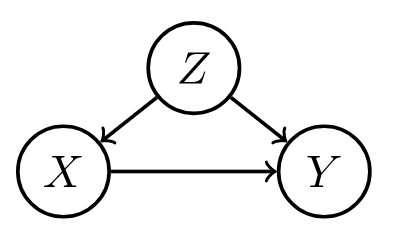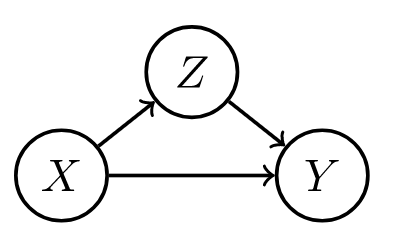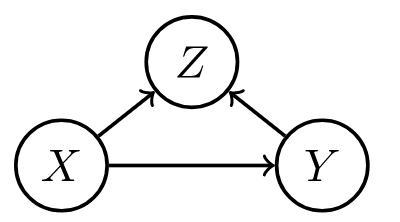confounder $z$, can be adjusted for confounder can vary causal effect conditioning on confounder z can explain away a cause
• structural causal model (scm) gives a set of variables $X_1, … X_i$ and and assignments of the form $X_i := f_i(X_{parents(i)}, \epsilon_i)$, which tell how to compute value of each node given parents
• exogenous variables $\epsilon_i$ = noise variables = disturbances = errors - node in the network that represents all the data not collected
• are not influenced by other variables
• modeler decides to keep these unexplained
• not the same as the noise term in a regression - need not be uncorrelated with regressors
• direct causes = parent nodes
• the $:=$ notation signifies a direction
• controlling for a variable (when we have a causal graph):
•  $P(Y=y do(T:=t)) = \sum_z \underbrace{P(Y=y T=t, T_{parents}=z)}{\text{effect for slice}} \underbrace{P(T{parents}=z)}_{\text{weight for slice}}$
•  post-intervention distribution $P(z, y do(t_0))$ - result of setting $T = t_0$
• also called “controlled” or “experimental” distr.
• counterfactual $Y^{T=t}(x)$ under SCM can be explicitly computed
• given structural causal model M, observed event x, action T:=t, target variable Y, define counterfactual $Y^{T=t}(x)$ in 3 steps:
• abduction - adjust noise variables to be consistent with $x$
• action - perform do-intervention, setting $T=t$
• prediction - compute target counterfactual, which follows directly from $M_t$, model where action has been performed
• counterfactuals can be derived from the model, unlike potential outcomes framework where counterfactuals are taken as primitives (e.g. they are undefined quantities which other quantities are derived from)
• identifiability
• effects are identifiable whenever model is Markovian
• graph is acyclic
• error terms are jointly independent
• in general, parents of $X$ are the only variables that need to be measured to estimate the causal effects of $X$
•  in this simple case, $E(Y do(x_0)) = E(Y X=x_0)$
• can plug into the algebra of an SEM to see the effect of intervention
• alternatively, can factorize the graph, set $x=x_0$ and then marginalize over all variables not in the quantity we want to estimate
• this is what people commonly call “adjusting for confounders”
• this is the same as the G-computation formula (Robins, 1987), which was derived from a more complext set of assumptions
• rules of do-calculus allow us to identify causal effects in general (e.g. in front-door adjustement)
• general criterion
• back-door / front-door
•  unconfounded children criterion: if it is possible to block all backdoor paths from $T$ to all of its children that are ancestors of $Y$ with a single conditioning set $S$, then $P(Y=y do(T=t))$ is identifiable (tian & pearl, 2002)
• examples
• ex. correlate flips
•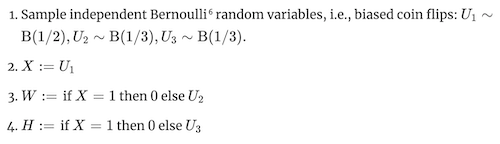• in this ex, $W$ and $H$ are usually correlated, so conditional distrs. are similar, but do operator of changing $W$ has no effect on $H$ (and vice versa)
•  notation: $P(H do(W:=1))$ or $P_{M[W:=1]}(h)$
•  ATE of $W$ on $H$ would be $P(H do(W:=1)) - P(H do(W:=0))$
• ex. simple structure
•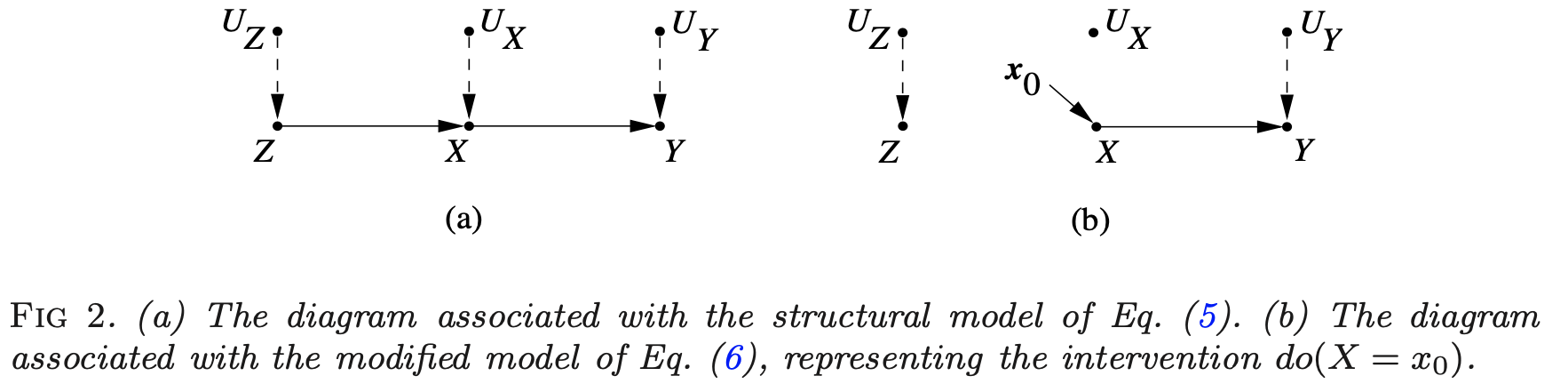where \begin{align}z &= f_Z(u_Z)\x&=f_X(z, u_X)\y&=f_Y(x, u_Y) \end{align}

# randomized experiments

Randomized experiments randomly assign a treatment, thus creating comparable treatment and control groups on average (i.e. controlling for any confounders).

## design

### stratification / matched-pairs design

• sometimes we want different strata, ex. on feature $X_i \in {1, …, K }$
• fully random experiment will not put same amount of points in each stratum + will have different treatment/control balance in each stratum
• stratification at design stage: stratify and run RCT for each stratum
• can do all the same fisher tests, usually by computing statistics within each stratum than aggregating
• sometimes bridge strata with global data
• ex. aligned rank statistic - normalize each $Y_i$ with stratum mean, then look at ranks across all data (hodges & lehmann 1962)
• can do neyman-rubin analysis as well - CLT holds with a few large strata and many small strata
• can prove that variance for stratified estimator is smaller when stratification is predictive of outcome

matched-pairs design - like stratification, but with only one point in each stratum (i.e. a pair)

• in each pair, one unit is given treatment and the other is not
• Fisherian inference
• $H_{0F}: Y_{i, j}^{T=1}=Y_{i, j}^{T=0} \; \underbrace{\forall i}{\text{all pairs}} \underbrace{\forall j}{\text{both units in pair}}$
• $\hat \tau_i = \underbrace{(2T_i - 1)}{-1\text{ or } 1}(Y{i1} - Y_{i2})$, where $T_i$ determines which of the two units in the pair was given treatment
• many other statistics…e.g. wilcoxon sign-rank statistic, sign statistic, mcnemar’s statistic
• Neymanian inference
• $\hat \tau = \frac 1 n \sum_i \tau_i$
• $\hat V = \frac 1 {n(n-1)} \sum_i (\hat \tau_i - \hat \tau)^2$
• can’t estimate variance as in stratified randomized experiment
• heuristically, matched-pairs design helps when matching is well-done and covariates are predictive of outcome (can’t check this at design stage though)
• can also perform covariate adjustment (on covariates that weren’t used for matching, or in case matching was inexact)

### rerandomization balances covariates

rerandomization adjusts for covariate imbalance given covariate vector $\mathbf x$ (assume mean 0) at design stage

• covariate difference-in-means: $\mathbf{\hat \tau_x} = \frac 1 {n_1} \sum_i T_i \mathbf x_i - \frac 1 {n_0} \sum_i (1 - T_i)\mathbf x_i$
• this is for RCT
• asymptotically zero, but real value need not be
• $\textbf{cov}(\hat\tau_x) = \frac 1 {n_1} S_x^2 + \frac 1 {n_0}S_x^2 = \frac{n}{n_1 n_0}S_x^2$
• $M = \hat \tau_x^T \textbf{cov}(\hat \tau_x)^{-1} \hat \tau_x$ - Mahalanabois distance measures difference between treatment/control groups
• invariant to non-degenerate linear transformations of $\mathbf x$
• can use other covariate balance criteria
• rerandomization: discard treatment allocations when $M \leq m_{thresh}$
• $m_{thresh}=\infty$: RCT
• $m_{thresh}=0$: few possible treatment allocations - limits randomness; in practice try to choose very small $m_{thresh}$
• proposed by Cox (1982) + Morgan & Rubin (2012)
• can derive asymptotic distr. for $\hat \tau$ (li, deng & rubin 2018)

• combining rerandomization + regression adjustment can achieve better results (li & ding, 2020)

## post-hoc analysis

### statification / matching

• post-stratification at analysis stage: condition on stratum
• can do conditional FRT or post-stratified Neymanian analysis
• can often improve efficiency
• is limited, because eventually there are no points in certain strata
• this is also called aggregating estimators (e.g. aggregating difference-in-means estimators)
• for continuous confounder, can also stratify on propensity score

• regression adjustment at analysis stage account for covariates x
• fisher random trial adjustment - 2 strategies
1. construct test-statistic based on residuals of statistical models
• regress $Y_i$ on $\mathbf x_i$ to obtain residual $e_i$ - then treat $e_i$ as pseudo outcome to construct test statistics
2. use regression coefficient as a test statistic
• regress $Y_i$ on $(T_i, \mathbf x_i$) to obtain coefficient of $T_i$ as the test statistic (Fisher’s ANCOVA estimator): $\hat \tau_F$
• Freedman (2008) found that this estimator had issues: biased, large variance, etc.
• Lin (2013) finds favorable properties of the $\hat \tau_F$ estimator
• can get minor improvements by instead using coefficient of $T_i$ in the OLS of $Y_i$ on $(T_i, \mathbf x_i, T_i \times \mathbf x_i$)

# quasi-experiments

Can also be called pseudo-experiments. These don’t explicitly randomize treatment, as in RCTs, but some property of the data allows us to control for confounders.

## natural experiments

• hard to justify
• e.g. jon snow on cholera - something acts as if it were randomized

## back-door criterion: capture parents of T

• back-door criterion - establishes if variables $T$ and $Y$ are confounded in a graph given set $S$
• sufficient set $S$ = admissible set - set of factors to calculate causal effect of $T$ on $Y$ requires 2 conditions - no element of $S$ is a descendant of $T$ - $S$ blocks all paths from $T$ to $Y$ that end with an arrow pointing to $T$ (i.e. back-door paths)
• intuitively, paths which point to $T$ are confounders
• if $S$ is sufficient set, in each stratum of $S$, risk difference is equal to causal effect
•  e.g. risk difference $P(Y=1 T=1, S=s) - P(Y=1 T=0, S=s)$
•  e.g. causal effect $P(Y=1 do(T=1), S=s) - P(Y=1 do(T=0), S=s)$
•  average outcome under treatment, $P(Y=y do(T=t)) = \sum_s P(Y=y T=t, S=s) P(S=s)$
• sometimes called back-door adjustment - really just covariate adjustment (same as we had in potential outcomes)
• there can be many sufficient sets, so we may choose the set which minimizes measurement cost or sampling variability
• propensity score methods help us better estimate the RHS of this average outcome, but can’t overcome the necessity of the back-door criterion

## front-door criterion: good mediator variable

• front-door criterion - establishes if variables $T$ and $Y$ are confounded in a graph given mediators $M$
• intuition: we have unknown confounder $U$, but can find a mediator $M$ between $T$ and $Y$ unaffected by $U$, we can still calculate the effect
• this is because we can calculate effect of $T$ on $M$, $M$ on $Y$, then multiply them
• $M$ must satisfy 3 conditions
• $M$ intercepts all directed paths from $T$ to $Y$ (i.e. front-door paths)
• there is no unblocked back-door path from $T$ to $M$
• all back-door paths from $M$ to $Y$ are blocked by $T$
•  when satisfied and $P(t, m) > 0$, then causal effect is identifiable as $P(y do(T=t)) = \sum_m P(m t) \sum_{t’} P(y t’, m) P(t’)$
• smoking ex. (hard to come up with many)
• $T$ = whether one smokes
• $Y$ = lung cancer
• $M$ = accumulation of tar in lungs
• $U$ = condition of one’s environment
• only really need to know about treatment, M, and outcome
graph LR
U -->Y
U --> T
T --> M
M --> Y


## regression discontinuity: running variable

• dates back to thistlethwaite & campbell, 1960
• treatment definition (e.g. high-school acceptance) has an arbitrary threshold (e.g. score on a test)
• comparing groups very close to the cutoff should basically control for confounding
•  easily satisfies unconfounding, but has no overlap at all ($P(T_i=1 Z_i=z)$ is always 0 or 1)
• $\tau_{c}=\mathbb{E}\left[Y_{i}(1)-Y_{i}(0) \mid Z_{i}=c\right]$ is identified via $\tau_{c}=\lim {z \downarrow c} \mathbb{E}\left[Y{i} \mid Z_{i}=z\right]-\lim {z \uparrow c} \mathbb{E}\left[Y{i} \mid Z_{i}=z\right]$ under continuity assumptions
• can estimate via local linear regr. – kernel fits things on either side
• needs assumptions on the smoothness of the mean function
• alternatively, assume some unconfounded noise in the running variable (i.e. the variable being thresholded)

## difference-in-difference

• difference-in-difference is a name given to many methods for estimating effects in longitudinal data = panel data
• requires data from both groups at 2 or more time periods (at least one before treatment and one after)
• simple constant-treatment model: $Y_{i t}=Y_{i t}(0)+T_{i t} \tau,$ for all $i=1, \ldots, n, t=1, \ldots$
• assumes no effect heterogeneity
• assumes treatment at time only effects outcomes at time t - this can be weird
• one approach for estimation: assume two-way model: $Y_{i t}=\alpha_{i}+\beta_{t}+T_{i t} \tau+\varepsilon_{i t}, \quad \mathbb{E}[\varepsilon \mid \alpha, \beta, T]=0$
• estimator for difference-in-difference with two timepoints
•  $\hat{\tau}=\frac{1}{\left \left{i: T_{i 2}=1\right}\right } \underbrace{\sum_{\left{i: T_{i 2}=1\right}}\left(Y_{i 2}-Y_{i 1}\right)}_{\text{diff for treated group}}-\frac{1}{\left \left{i: T_{i 2}=0\right}\right } \underbrace{\sum_{\left{i: T_{i 2}=0\right}}\left(Y_{i 2}-Y_{i 1}\right)}_{\text{diff for untreated group}}$
• second approach for estimation of constant-treatment: interactive panel models $Y_{i t}=A_{i .} B_{t .}^{\prime}+T_{i t} \tau+\varepsilon_{i t}, \quad \mathbb{E}[\varepsilon \mid A, B, T]=0, \quad A \in \mathbb{R}^{n \times k}, B \in \mathbb{R}^{T \times k}$
• allows for rank $k$ matrix, less restrictive (no longer forces parallel trends for all units)
• can estimate with synthetic controls (abadie, diamon, & hainmueller, 2010)
• artificially re-weight unexposed units (i.e. units with $T_i=0$) so their average trend matches the unweighted mean trend up to time $t_0$
• if the weights create thes parallel trends, they should alos balance the latent factors $A_i$
•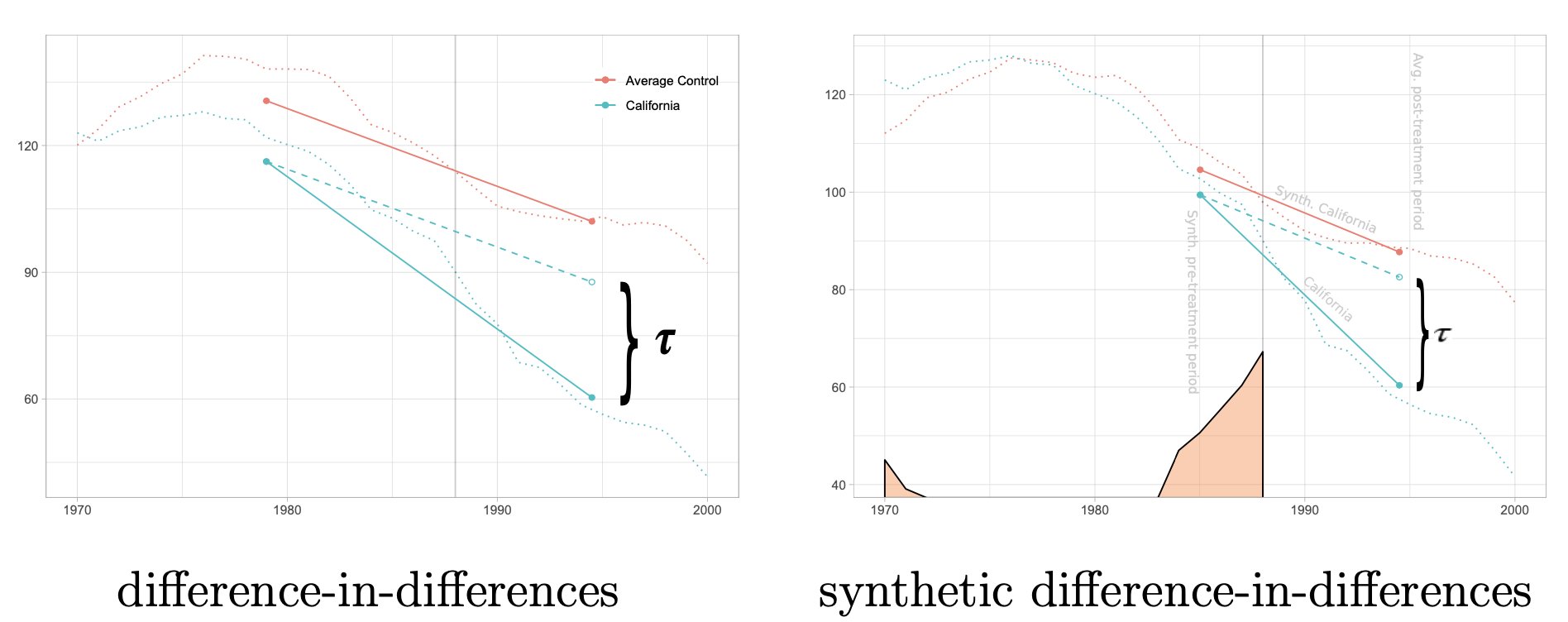• trends are clearly not parallel, but after reweighting they become parallel
• many other estimators, e.g. via clustering or nuclear norm minimization
• third approach: design-based assumptions e.g. $Y_{i .}(0) \perp T_{i .} \mid S_{i}, \quad S_{i}=\sum_{t=1}^{T} T_{i t}$
• ex. $Y_{it}$ is health outcome, $T_{it}$ is medical treatment, $S_i$ is unobserved health-seeking behavior
• $\hat{\tau}=\sum_{i, t} \gamma_{i t} Y_{i t}$ where $\gamma$-matrix depends only on treatment assignment

## instrumental variable

• graph LR
I --> T
X --> T
T --> Y

• instrument $I$- measurable quantity that correlates with the treatment, and is $\underbrace{\color{NavyBlue}{\text{only related to the outcome via the treatment}}}_{\textbf{exclusion restriction}}$

• precisely 3 conditions must hold for $I_i$:

• exogenous: $\varepsilon_{i} \perp I_{i}$
• relevant: $\operatorname{Cov}\left[T_{i}, I_{i}\right] \neq 0$
• exclusion restriction: any effect of $I_{i}$ on $Y_{i}$ must be mediated via $T_{i}$
• uncheckable
• intuitively, need to combine effect of instrument on treatment and effect of instrument on outcome (through treatment)

• in practice, often implemented in a 2-stage least squares (regress $I \to T$ then $T\to Y$)
• $Y_{i}=\alpha+T_{i} \tau+\varepsilon_{i}, \quad \varepsilon_{i} \perp I_{i}$
• $T_{i}=I_{i} \gamma+\eta_{i}$
• most important point is that $\epsilon_i \perp I_i$
• Wald estimator = $\frac{Cov(Y, I)}{Cov(T, I)}$
• LATE = local average treatment effect - this estimate is only valid for the patients who were influenced by the instrument
• we may have many potential instruments
• in this case, can learn a funciton of the instruments via cross-fitting as an instrument
• examples

• $I$: stormy weather, $T$ price of fish, $Y$ demand for fish
• stormy weather makes it harder to fish, raising price but not affecting demand
• $I$: quarter of birth, $T$: schooling in years, $Y$: earnings (angrist & krueger, 1991)
• $I$: sibling sex composition, $T$: family size, $Y$: mother’s employment (angist & evans, 1998)
• $I$: lottery number, $T$: veteran status, $Y$: mortality
• $I$: geographic variation in college proximity, $T$: schooling, $Y$: wage (card, 1993)

### effect under non-compliance

• CACE $\tau_c$ (complier average causal effect) = LATE (local average treatement effect)
• technical setting: noncompliance - sometimes treatment assigned $I$ and treatment received $T$ are different

• assumptions
• randomization = instrumental unconfoundedness: $I \perp {T^{I=1}, T^{I=0}, Y^{I=1}, Y^{I=0} }$
• randomization lets us identify ATE of $I$ on $T$ and $I$ on $Y$
• $\tau_{T}=E{T^{I=1}-T^{I=0}}=E(T \mid I=1)-E(T \mid I=0)$
• $\tau_{Y}=E{Y^{I=1}-Y^{I=0}}=E(Y \mid I=1)-E(Y \mid I=0)$
• intention-to-treat $\tau_Y$
• four possible outcomes:
• $C_{i}=\left{\begin{array}{ll} \mathrm{a}, & \text { if } T_{i}^{I=1}=1 \text { and } T_{i}^{I=0}=1 \text{ always taker} \mathrm{c}, & \text { if } T_{i}^{I=1}=1 \text { and } T_{i}^{I=0}=0\text{ complier} \mathrm{d}, & \text { if } T_{i}^{I=1}=0 \text { and } T_{i}^{I=0}=1 \text{ defier} \mathrm{n}, & \text { if } T_{i}^{I=1}=0 \text { and } T_{i}^{I=0}=0\text{ never taker} \end{array}\right.$
• exclusion restriction: $Y_i^{I=1} = Y_i^{I=0}$ for always-takers and never-takers
• means treatment assignment affects outcome only if it affects the treatment
• monotonicity: $P(C=d) = 0$ or $T_i^{U=1} \geq T_i^{U=0} \; \forall i$ - there are no defiers
•  testable implication: $P(T=1 I=1) \geq P(T=1 C=0)$
• under these 3 assumptions, LATE $\tau_c = \frac{\tau_Y}{\tau_T} = \frac{E(Y \mid I=1)-E(Y \mid I=0)}{E(T \mid I=1)-E(T \mid I=0)}$

• this can be estimated with the WALD estimator
• basically just scales the intention-to-treat estimator, so usually similar conclusions
• if we have some confounders $X$, can adjust for them
• the smaller $\tau_T$ is (i.e. the more noncompliance there is), the worse properties the LATE estimator has
•  instrumental variable inequalities $E(Q I=1) \geq E(Q I=0)$ where $Q = TY, T(1-Y), (1-T)Y, T+Y - TY$
•  instrumental inequality (Pearl 1995) - a necessary condition for any variable $I$ to qualify as an instrument relative to the pair $(T, Y)$: $\max_T \sum_Y \left[ \max_I P(T,Y I) \right] \leq 1$
• when all vars are binary, this reduces to the following:
• $P(Y=0, T=0 \mid I=0) + P(Y=1, T=0 \mid I=1) \leq 1$ $P(Y=0, T=1 \mid I=0)+P(Y=1, T=1 \mid I=1) \leq 1$ $P(Y=1, T=0 \mid I=0)+P(Y=0, T=0 \mid I=1) \leq 1$ $P(Y=1, T=1 \mid I=0)+P(Y=0, T=1 \mid I=1) \leq 1$
•  instrumental variable criterion - sufficient condition for identifying the causal effect $P(y do(t))$ is that every path between $T$ and any of its children traces at least one arrow emanating from a measured variable
• sometimes this is satisfied even when back-door criterion is not

# observational analysis

The emphasis in this section is on ATE estimation, as an example of the considerations required for making causal conclusions. Observational analysis focuses on adjusting for observed confounding.

## ATE estimation basics

• assume we are given iid samples of ${ X_i, T_i, Y_i^{T=1}, Y_i^{T=0} }$, and drop the index $i$
• $\tau = E{Y^{T=1} - Y^{T=0}}$: average treatment effect (ATE) - goal is to estimate this
•  $\tau_T =E{Y^{T=1}−Y^{T=0} T =1}$: ATE on the treated units
•  $\tau_C =E{Y^{T=1}−Y^{T=0} T =0}$: ATE on the control units
•  $\tau_{PF} = E[Y T=1] - E[Y T=0]$: prima facie causal effect
•  $= E[Y^{T=1} T=1] - E[Y^{T=0} T=0]$
• naive, but computable!
• generally biased, with selection biases:
•  $E[Y^{T=0} T=\textcolor{NavyBlue}1] - E[Y^{T=0} T=\textcolor{NavyBlue}0]$
•  $E[Y^{T=1} T=\textcolor{NavyBlue}1] - E[Y^{T=1} T=\textcolor{NavyBlue}0]$
• randomization tells us the treatment is independent of the outcome with/without treatment $T \perp {Y^{T=1}, Y^{T=0}}$, so the selection biases are zero (rubin, 1978)
• $\implies \tau = \tau_T = \tau_C$
• this is more important than balancing the distr. of covariates
• conditioning on observed X, selection bias terms are zero:
•  $E{Y^{T=0} T=1, X} = E{Y^{T=0} T=0, X}$
•  $E{Y^{T=1} T=1, X} = E{Y^{T=1} T=0, X}$
• $\implies \tau(X) = \tau_T(X) = \tau_C(X) = \tau_{PF}(X)$
• ex. stratified ATE estimator (given discrete covariate)

• ATE conditional outcome modeling (all assume ignorability)
• ex. $\tau = \beta_t$ in OLS
•  $E(Y T, X) = \beta_0 + \beta_t T + \beta_x^TX$
• assumes treatment effect is same for all individuals
• ex. $\tau = \beta_t + \beta_{tx}^TE(X)$
•  $E(Y T, X) = \beta_0 + \beta_tT + \beta_x^TX + \beta^T_{tx} X T$
• incorporates heterogeneity
• intuition

• much like imputing missing potential outcomes using a linear model
• using nearest-neighbor regr. would correspond to a matching-without-replacement estimator
• sometimes use propensity scores as a predictor as well
• assuming linear form is relatively strong assumption compared to that made by weighting / stratification

• these easily generalize to when $T$ is continuous

• $\hat \tau = \frac 1 n \sum_i (\hat \mu_1(X_i) - \hat \mu_0(X_i))$
•  general mean functions $\hat \mu_1(x), \hat \mu_0(x)$ approximate $\mu_i =E{ Y^{T=i} X}$
• consistent when $\mu_i$ functions are well-specified
• M-bias
• originally, $T \perp Y$ 😊
• after adjusting for X, $T \not \perp Y$ 🙁
• graph LR
U1 --> T
U1 --> X
U2 --> X
U2 --> Y

• Z-bias: after adjusting, bias is larger
•   graph LR
X --> T
T --> Y
U --> Y
U --> T


## weighting methods

Weighting methods assign a different importance weight to each unit to match the covariates distributions across treatment groups after reweighting. Balance is often used as a goodness of fit check after weighting (imbens & rubin 2015).

### inverse propensity weighting

•  propensity score $e(X, Y^{T=1}, Y^{T=0}) = P{T=1 X, Y^{T=1}, Y^{T=0}}$
•  under strong ignorability, $e(X)=P(T=1 X)$
•  Thm. if $\underbrace{T \perp { Y^{T=1}, Y^{T=0}} \color{NavyBlue} X}_{\text{strong ignorability on X}}$, then $\underbrace{T \perp { Y^{T=1}, Y^{T=0}} \textcolor{NavyBlue}{e(X)}}_{\text{strong ignorability on e(X)}}$
• therefore, can stratify on $e(X)$, but still need to estimate $e(X)$, maybe bin it into K quantiles (and pick K)
• could combine this propensity score weighting with regression adjustment (e.g. within each stratum)
• assumes positivity
• intuition: $e(X)$ fully mediates path from $X$ to $T$
•  Thm. $T \perp X \; \; e(X)$. Moreover, for any function $h$, $E{\frac{T \cdot h(X)}{e(X)}} = E{\frac{(1-T)h(X)}{1-e(X)}}$
• can use this result to check for covariate balance in design stage
• can view $h(X)$ as psuedo outcome and estimtate ATE
• if we specify it to something like $h(X)=X$, then it should be close to 0
• $\hat \tau_{ht} = \frac 1 n \sum_i \frac{T_iY_i}{\hat e(X_i)} - \frac 1 n \sum_i \frac{(1-T_i)Y_i}{1-\hat e(X_i)}$ = inverse propensity score weighting estimator = horvitz-thompson estimator (horvitz & thompson, 1952)
• weight outcomes by $1/e(X)$ for treated individuals and $1/(1-e(X))$ for untreated
•  Based on Thm. if $\underbrace{T \perp { Y^{T=1}, Y^{T=0}} X}_{\text{strong ignorability on X}}$, then:
• $E{Y^{T=1}} = E \left { \frac{TY}{e(X)} \right }$
• $E{Y^{T=0}} = E\left { \frac{(1-T)Y}{1-e(X)} \right }$
• $\implies \tau = E \left { \frac{TY}{e(X)} - \frac{(1-T)Y}{1-e(X)} \right }$
• consistent when propensity scores are correctly specified
• intuition: assume we have 2 subgroups in our data
• if prob. of a sample being assigned treatment in one subgroup is low, should upweight it because this sample is rare + likely gives us more information
• this also helps balance the distribution of the treatment for each subgroup
• if we add a constant to $Y$, then this estimator changes (not good) - if we adjust to avoid this change, we get the Hajek estimator (hajek, 1971), which is often more stable
• scores near 0/1 are unstable - sometimes truncate (“trim”) or drop units with these scores
• fundamental problem is ovelap of covariate distrs. in treatment/control
• when score = 0 or 1, counterfactuals may not even be well defined
• stratified estimator can be seen as a particular case of IPW estimator

### doubly robust estimator

• combines weighting and regr. adjustment
• $\hat \tau^{\text{dr}} = \hat \mu_1^{dr} - \hat \mu_0^{dr}$ = doubly robust estimator = augmented inverse propensity score weighting estimator (robins, rotnizky, & zhao 1994, scharfstein et al. 1999, bang & robins 2005)
• given $\mu_1(X, \beta_1)$, $\mu_0(X, \beta_0)$, e.g. linear
• given $e(X, \alpha)$, e.g. logistic
• $\tilde{\mu}{1}^{\mathrm{dr}} =E\left[ \overbrace{\mu{1}\left(X, \beta_{1}\right)}^{\text{outcome mean}} + \overbrace{\frac{T\left{Y-\mu_{1}\left(X, \beta_{1}\right)\right}}{e(X, \alpha)}}^{\text{inv-prop residuals}}\right]$
• $\tilde{\mu}{0}^{\mathrm{dr}} =E\left[\mu{0}\left(X, \beta_{0}\right) + \frac{(1-T)\left{Y-\mu_{0}\left(X, \beta_{0}\right)\right}}{1-e(X, \alpha)}\right]$
• augments the oucome regression mean with inverse-propensity of residuals
• can alternatively augment inv propensity score weighting estimator by the outcome models:
• \begin{aligned} \tilde{\mu}{1}^{\mathrm{dr}} &=E\left[\frac{T Y}{e(X, \alpha)}-\frac{T-e(X, \alpha)}{e(X, \alpha)} \mu{1}\left(X, \beta_{1}\right)\right] \tilde{\mu}{0}^{\mathrm{dr}} &=E\left[\frac{(1-T) Y}{1-e(X, \alpha)}-\frac{e(X, \alpha)-T}{1-e(X, \alpha)} \mu{0}\left(X, \beta_{0}\right)\right] \end{aligned}
• consistent if either the propensity scores or mean functions are well-specified:
• propensities well-specified: $e(X, \alpha) = e(X)$
• mean functions well-specified: $\left{\mu_{1}\left(X, \beta_{1}\right)=\mu_{1}(X), \mu_{0}\left(X, \beta_{0}\right)=\mu_{0}(X)\right}$
• in practice, often use cross-fitting (split the data randomly into two halves $\mathcal I_1$ and $\mathcal I _2$)
•  $\hat{\tau}_{A I P W}=\frac{\left \mathcal{I}_{1}\right }{n} \hat{\tau}^{\mathcal{I}_{1}}+\frac{\left \mathcal{I}_{2}\right }{n} \hat{\tau}^{\mathcal{I}_{2}}$
•  $\hat{\tau}^{\mathcal{I}_{1}}=\frac{1}{\left \mathcal{I}_{1}\right } \sum_{i \in \mathcal{I}{1}}\left(\hat{\mu}{(1)}^{\mathcal{I}{2}}\left(X{i}\right)-\hat{\mu}{(0)}^{\mathcal{I}{2}}\left(X_{i}\right)\right. \left.+W_{i} \frac{Y_{i}-\hat{\mu}{(1)}^{\mathcal{I}{2}}\left(X_{i}\right)}{\hat{e}^{\mathcal{I}{2}}\left(X{i}\right)}-\left(1-W_{i}\right) \frac{Y_{i}-\hat{\mu}{(0)}^{\mathcal{I}{2}}\left(X_{i}\right)}{1-\hat{e}^{\mathcal{I}{2}}\left(X{i}\right)}\right)$
• avoids bias due to overfitting
• allows us to ignore form of estimators $\hat \mu$ and $\hat e$ and depend only on overlap, consistency, and risk decay (so CV risk of estimators should be small)
• targeted maximum likelihood (van der laan & rubin, 2006) - more general than DRE

### alternative weighting

• solutions to deal with extreme weights (from assaad et al. 2020):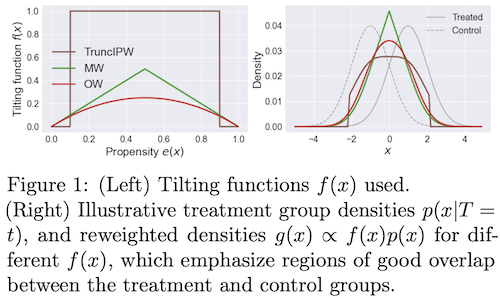• Matching Weights (Li & Greene, 2013): MW
• Truncated IPW (Crump et al., 2009): TruncIPW
• Overlap Weights (Li et al., 2018): OW - this uses (1 - IPW weights), and doesn’t suffer from instability at extreme values
• when estimating propensity scores with neural nets, often overconfident
• in general, there is a more general class of weights that can be used to balance covariates (li, morgan, & zaslavsky, 2016)
• directly incorporating covariate imbalance in weight construction (Graham et al., 2012; Diamond & Sekhon, 2013)
• unifying perspective on these methods via covariate-balancing loss functions (zhao, 2019)
• in general, balancing need not directly balance the propensity scores
• instead, might find propensity weights which balance covariates along certain basis functions $\psi_j(x)$
• $\frac{1}{n} \sum_{i=1}^{n} \frac{W_{i} \psi_{j}\left(X_{i}\right)}{\hat{e}\left(X_{i}\right)} \approx \frac{1}{n} \sum_{i=1}^{n} \psi_{j}\left(X_{i}\right),$ for all $j=1,2, \ldots$
• this can be desirable in high dims, when propensity scores may be unstable
• hard moment-matching conditions (Li & Fu, 2017; entropy balancing from Hainmueller, 2012; Imai & Ratkovic, 2014)
• soft moment-matching conditions (Zubizarreta, 2015)
• approximate residual balancing (athey, imbens, & wager, 2018) - combines balancing weights with a regularized regression adjustment for learning ATE from high-dimensional data
• Learning Causal Effects via Weighted Empirical Risk Minimization (jung et al. 2020) - estimate any computation (which usually requires actually modeling individual conditional probabilities) using weighted empirical risk minimization

## stratification / matching

Matching methods choose try to to equate (or “balance’’) the distribution of covariates in the treated and control groups by picking well-matched samples of the original treated and control groups

• Matching methods for causal inference: A review and a look forward (stuart 2010)
• matching basics
• includes 1:1 matching, weighting, or subclassification
• linear regression adjustment (so not matching) can actually increase bias in the estimated treatment effect when the true relationship between the covariate and outcome is even moderately non-linear, especially when there are large differences in the means and variances of the covariates in the treated and control groups
• matching distance measures
• propensity scores summarize all of the covariates into one scalar: the probability of being treated
• defined as the probability of being treated given the observed covariates
• propensity scores are balancing scores: At each value of the propensity score, the distribution of the covariates X defining the propensity score is the same in the treated and control groups – usually this is logistic regresion
• if treatment assignment is ignorable given the covariates, then treatment assignment is also ignorable given the propensity score: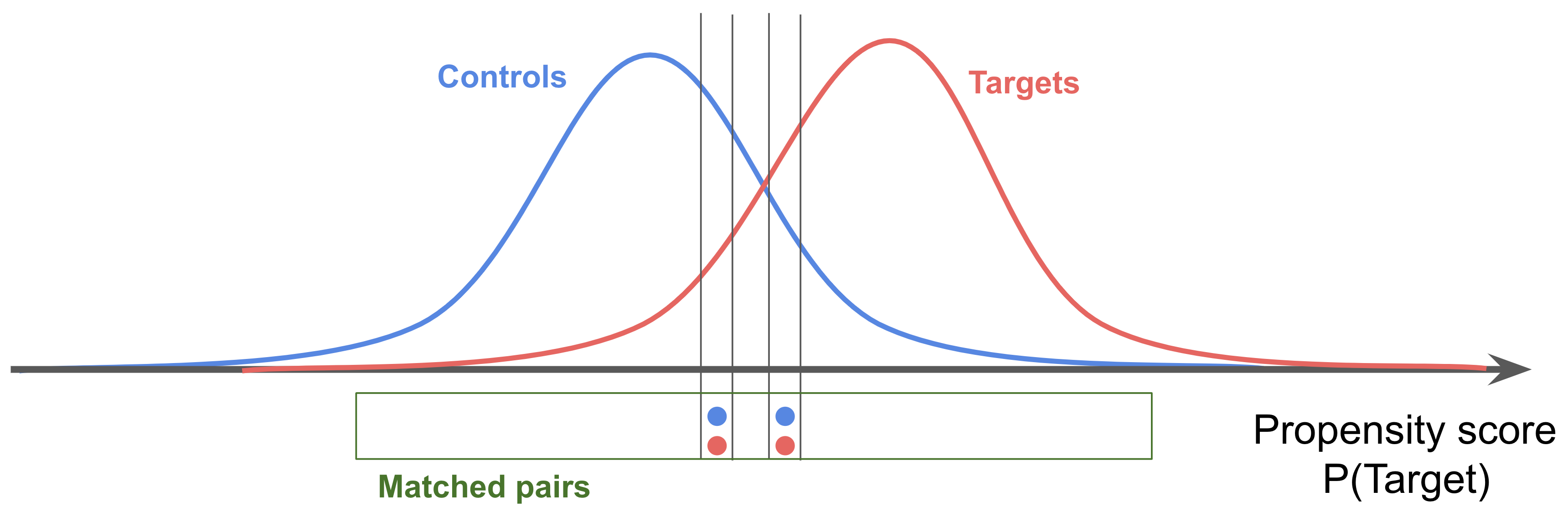• hard constraints are called “exact matching” - can be combined with other methods
• mahalanabois distance
• matching methods
• stratification = cross-tabulation - only compare samples when confounding variables have same value
• nearest-neighbor matching - we discard many samples this way (but samples are more similar, so still helpful)
• optimal matching - consider all potential matches at once, rather than one at a time
• ratio matching - could match many to one (especially for a rare group), although picking the number of matches can be tricky
• with/without replacement - with seems to have less bias, but more practical issues
• subclassification/weighting: use all the data - this is nice because we have more samples, but we also get some really poor matches
• subclassification - stratify score, like propensity score, into groups and measure effects among the groups
• full matching - automatically picks the number of groups
• weighting - use propensity score as weight in calculating ATE (also know as inverse probability of treatment weighting)
• common support - want to look at points which are similar, and need to be careful with how we treat points that violate similarity
• genetic matching - find the set of matches which minimize the discrepancy between the distribution of potential confounders
• diagnosing matches - are covariates balanced after matching?
• ideally we would look at all multi-dimensional histograms, but since we have few points we end up looking at 1-d summaries
• one standard metric is difference in means of each covariate, divided by its stddev in the whole dataset
• analysis of the outcome - can still use regression adjustment after doing the matching to clean up residual covariances
• unclear how to propagate variance from matching to outcome analysis
• matching does not scale well to higher dimensions (abadie & imbens, 2005), improving balance for some covariates at the expense of others
• bias-corrected matching estimator averages over all matches
• if we get perfect matches on covariates $X$ (or propensity score), it is just like doing matched design
• in practice, we only get approximate matches
•  for a unit, we take its value and impute its counterfactual as $\frac 1 { matches } \sum_{\text{match} \in {\text{matches}}} Y_{\text{match}}$
• requires complex bootstrap procedure to obtain variance
• bias-corrected matching estimator is very similar to doubly robust estimator
• \begin{aligned} \hat{\tau}^{\mathrm{mbc}} &=n^{-1} \sum_{i=1}^{n}\left{\hat{\mu}{1}\left(X{i}\right)-\hat{\mu}{0}\left(X{i}\right)\right}+n^{-1} \sum_{i=1}^{n}\left{\left(1+\frac{K_{i}}{M}\right) T_{i} \hat{R}{i}-\left(1+\frac{K{i}}{M}\right)\left(1-T_{i}\right) \hat{R}{i}\right} \hat{\tau}^{\mathrm{dr}} &=n^{-1} \sum {i=1}^{n}\left{\hat{\mu}{1}\left(X{i}\right)-\hat{\mu}{0}\left(X{i}\right)\right}+n^{-1} \sum_{i=1}^{n}\left{\frac{T_{i} \hat{R}{i}}{\hat{e}\left(X{i}\right)}-\frac{\left(1-T_{i}\right) \hat{R}{i}}{1-\hat{e}\left(X{i}\right)}\right} \end{aligned}

## misc methods

• double machine learning
• first
• fit a model to predict $Y$ from $X$ to get $\hat Y$
• fit a model to predict $T$ from $X$ to get $\hat T$
• next, predict $Y- \hat Y$ from $T - \hat T$, which has no in some sense removed effects of $X$
• ex. Chernozhukov et al. (2018), ‘Double/debiased machine learning for treatment and structural parameters’
• ex. Felton (2018), Chernozhukov et al. on Double / Debiased Machine Learning
• ex. Syrgkanis (2019), Orthogonal/Double Machine Learning
• ex. Foster and Syrgkanis (2019), Orthogonal Statistical Learning

# assumptions

## common assumptions

• unconfoundedness
• exchangeability $T \perp !!! \perp { Y^{t=1}, Y^{t=0}}$ = exogeneity = randomization: the value of the counterfactuals doesn’t change based on the choice of the treatment
• intuition: we could have exchanged the treatment groups and done this experiment
• there are weaker forms of this assumption, such as mean exchangeability (where only means are exchangeable)
•  ignorability $T \perp !!! \perp { Y^{T=1}, Y^{T=0}} X$ = unconfoundedness = selection on observables = conditional exchangeability
• very hard to check!
• rubin 1978; rosenbaum & rubin 1983
• this is strong ignorability, weak ignorability is a little weaker
• this will make selection biases zero
•  $E[Y^{T=0} T=\textcolor{NavyBlue}1] - E[Y^{T=0} T=\textcolor{NavyBlue}0]$
•  $E[Y^{T=1} T=\textcolor{NavyBlue}1] - E[Y^{T=1} T=\textcolor{NavyBlue}0]$
• graph assumptions
• back-door criterion, front-door criterion, unconfounded children criterion
• basics in a graph
• modularity assumptions
• markov assumptions
• exclusion restrictions: For every variable $Y$ having parents $PA(Y)$ and for every set of endogenous variables $S$ disjoint of $PA(Y)$, we have $Y^{PA(y)} = Y^{PA(Y), S}$
• fixing a variables parents fully determines it
• independence restrictions: variables are independent of noise variables given their markov blanket
• overlap / imbalance assumption
•  positivity $P(T=1 x) > 0 : \forall x$ = overlap = common support - probability of receiving any treatment is positive for every individual
• without overlap, assumption of linear effect can be very strong (e.g. extrapolates out of sample)
• strong overlap assumption: propensity scores are bounded away from 0 and 1
• $0 < \alpha_L \leq e(X) \leq \alpha_U < 1$
• when $e(X)=$0 or 1, potential outcomes are not even well-defined (king & zeng, 2006)
• things can be matched
• overlap and unconfoundedness trade off (e.g. see amour et al. 2020)
• strong overlap also implies moment bounds for covariate balance
• treatment assumptions
• treatment is not ambiguous
• no interference: my outcome is unaffected by anyone else’s treatment
• $Y_i(t_1, …,t_n) = Y_i(t_i)$
• consistency: $Y=Y^{t=0}(1-T) + Y^{t=1}T$ - outcome agrees with the potential outcome corresponding to the treatment indicator
• this grounds the definition of the counterfactuals $Y^{t=0}, Y^{t=1}$
• alternatively, $T=t \implies Y=Y(t)$
• basically means treatment is well-defined
• stable unit treatment value assumption (SUTVA) = no-interference assumption (Rubin, 1980): $Y_i = Y_i(T_i)$
• means unit $i$’s outcome is a function of unit $i$’s treatment
• no interference
• consistency (+deterministic potential outcomes)
• is it something that could be intervened on?
• can we intervene on something like Race? Soln: intervene on perceived race
• can we intervene on BMI? many potential interventions: e.g. diet, exercise
• SCM counterfactuals further assume 2 things:
• $Y_{yz} = y \quad \forall y, \text{ subsets }Z\text{, and values } z \text{ for } Z$
• ensures interventions $do(Y=y)$ results in $Y=y$, regardless of concurrent interventions, say $do(Z=z)$
• $X_z = x \implies Y_{xz} = Y_z \quad \forall x, \text{ subsets }Z\text{, and values } z \text{ for } Z$
• generalizes above
• ensures interventions $do(X=x)$ results in appropriate counterfactual, regardless of holding a variable fixed, say $Z=z$
• assumptions for ATE being identifiable: exchangeability (or ignorability) + consistency, positivity
• Independent Causal Mechanisms (ICM) Principle: The causal generative process of a system’s variables is composed of autonomous modules that do not inform or influence each other.
• In the probabilistic case, this means that the conditional distribution of each variable given its causes (i.e., its mechanism) does not inform or influence the other mechanisms.

## assumption checking

### check ignorability: use auxilary outcome

• negative outcome - assume we have a secondary informative outcome $Y’$

• $Y’$ is similar to $Y$ in terms of confounding: if we believe $T \perp Y(t) \mid X$ we also think $T \perp Y’(t) \mid X$

• we know the expected effect of $T$ on $Y’$ (ex. it should be 0)

• $\tau(T \to Y’) = E{Y’^{T=1} - Y’^{T=0})}$

• ex. cornfield et al. 1959 - effect of smoking on car accident
• graph LR
X(X) -->Y'(Y')
X --> Y
X --> T
T --> Y

• negative exposure - assume we have a secondary informative treatment $T’$
• $T’$ is similar to $T$ in terms of confounding: if we believe $T \perp Y(t) \mid X$ we also think $T’ \perp Y(t) \mid X$
• we know the expected effect of $T’$ on $Y$ (ex. it should be 0)
• $\tau(T’ \to Y) = E{Y^{T’=1} - Y^{T’=0}}$
• ex. maternal exposure $T$ and parental exposure $T’$
• graph LR
X -->T'
X --> Y
X --> T
T --> Y


### check ignorability impact: sensitivity analysis wrt unmeasured confounding

• sensitivity analysis measures how “sensitive” a model is to changes in the value of the parameters / structure / assumptions of the model

• we focus on sensitivity analyses wrt unmeasured confounding - how strong the effects of an unobserved covariate $U$ on the exposure and/or the outcome would have to be to change the study inference (estimated effect of $T$ on $Y$)

• there are many types of such analyses (for a review, see liu et al. 2013)

• ex. rosenbaum-type: interested in finding thresholds of the following association(s) that would render test statistic of the study inference insignificant

• e.g. true odds-ratio of outcome and treatment, adjusted for $X$ and $U$
• unobserved confounder and the treatment (odds-ratio $OR_{\text{UT}}$)
• and/or unobserved confounder and the outcome (odds-ratio $OR_{\text{UY}}$)
• usually used after matching
• 3 types
• primal sensitivity analysis: vary $OR_{\text{UT}}$ with $OR_{\text{UY}} = \infty$
• dual sensitivity analysis: vary $OR_{\text{UY}}$ with $OR_{\text{UT}}=\infty$
• simultaneous sensitivity analysis: vary both vary $OR_{\text{UT}}$ and $OR_{\text{UY}}$
• ex. confidence-interval methods: quantify unobserved confounder under specifications then arrive at target of interest + confidence interval, adjusted for $U$
• first approach: use association between $T, Y, U$ to create data as if $U$ was observed
•  specifications: $P(U T=0$), $P(U T=1)$ (greenland, 1996)
• specifications: $OR_{\text{UY}}$ and $OR_{\text{UT}}$, $U$ evenly distributed (harding, 2003)
•  given either of these specifications, can fill out the table of $X, Y U$, then use these weights to re-create data and fit weighted logistic regression
• second approach: use association between $T, Y, U$ to compute adjustment
• this approach can relax some assumptions, such as the no-three-way-interaction assumption
•  specifications: $P(U T=1), P(U T=0), OR_{\text{UY T=1}}, OR_{\text{UY T=0}}$(lin et al. 1998)
•  specifications: $P(U T=1), P(U T=0), OR_{\text{UY}}$ (vanderweele & arah, 2011)
• ex. cornfield-type (cornfield et al. 1959) - seminal work
•  ignorability does not hold $T \not \perp { Y^{T=1}, Y^{T=0}} X$
•  latent ignorability holds $T \perp { Y^{T=1}, Y^{T=0}} (X, U)$
• true causal effect (risk ratio): $\mathrm{RR}_{x}^{\text {true }}=\frac{\operatorname{pr}{Y^{T=1}=1 \mid X=x}}{\operatorname{pr}{Y^{T=0}=1 \mid X=x}}$
• observed: $\mathrm{RR}_{x}^{\mathrm{obs}}=\frac{\operatorname{pr}(Y=1 \mid T=1, X=x)}{\operatorname{pr}(Y=1 \mid T=0, X=x)}$
• we can ask how strong functions of U, T, Y all given X must be to explain away an observed association
• $\mathrm{RR}_{T U \mid x}=\frac{\operatorname{pr}(U=1 \mid T=1, X=x)}{\operatorname{pr}(U=1 \mid T=0, X=x)}$
• $\mathrm{RR}_{U Y \mid x}=\frac{\operatorname{pr}(Y=1 \mid U=1, X=x)}{\operatorname{pr}(Y=1 \mid U=0, X=x)}$
• Thm: under latent ignorability, $\mathrm{RR}{x}^{\mathrm{obs}} \leq \frac{\mathrm{R} \mathrm{R}{T U \mid x} \mathrm{RR}{U Y \mid x}}{\mathrm{RR}{T U \mid x}+\mathrm{RR}_{U Y \mid x}-1}$
• ex. bounds on direct effects with confounded intermediate variables (Cai et al. 2008)

• bounds with no assumptions (manski 1990)
• worst-case bounds are very poor
• also bounds with monotone treatment response (manski, 1997)
• bounds with optimal treatment selection (i.e. individuals always receive the treatement that is best for them) (manski, 1990, “nonparametric bounds on treatment effects”)
• Sensitivity Analysis in Observational Research: Introducing the E-Value (vanderweele & ding, 2017)

• E-value = min strength of association (risk ratio) that an unmeasured confounder would require with both $T$ and $Y$ to explain away a specific treatment-outcome association, conditional on $X$
• higher = more causal
• Interpretable Sensitivity Analysis for Balancing Weights (soriano et al. 2021) - percentile bootstrap procedure applied to balancing weights estimators